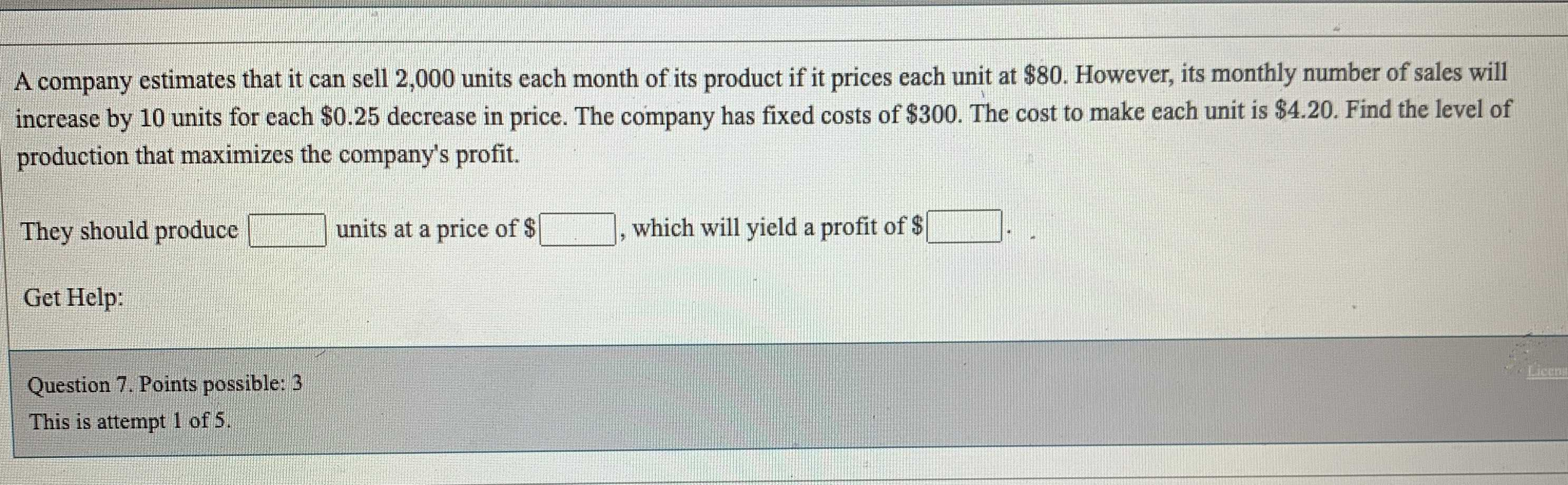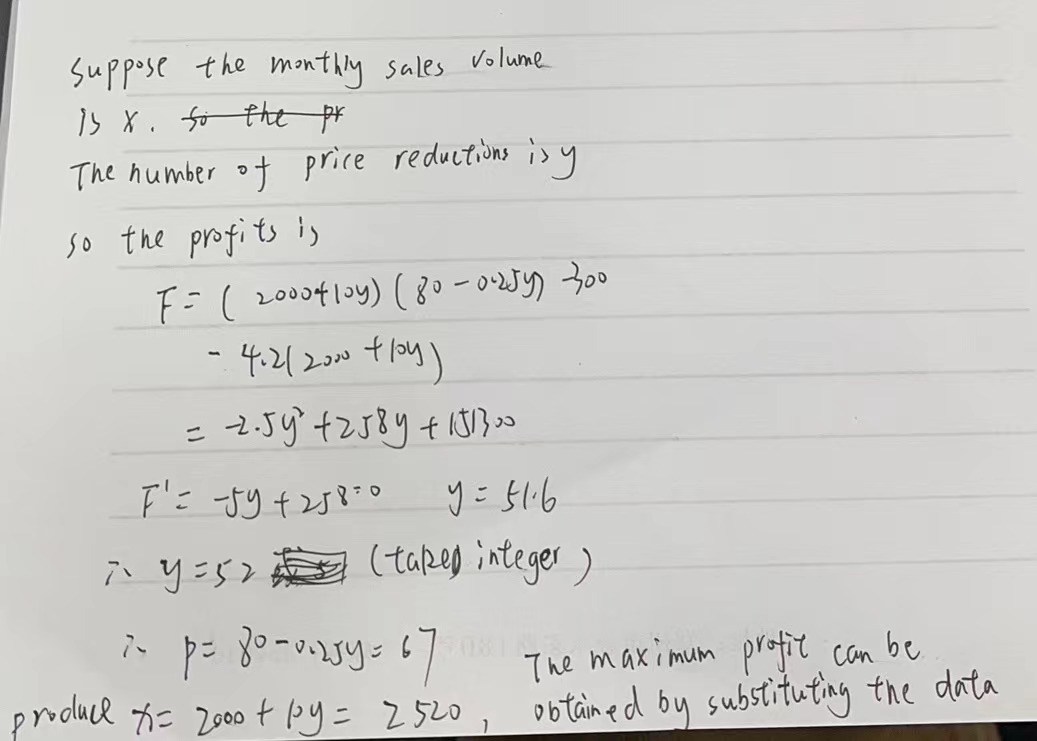### ¿Todavía tienes preguntas de matemáticas?

Pregunte a nuestros tutores expertos
Algebra
PreguntaA company estimates that it can sell $$2,000$$ units each month of its product if it prices each unit at $$\ 80$$ . However, its monthly number of sales will increase by $$10$$ units for each $$\ 0.25$$ decrease in price. The company has fixed costs of $$\ 300$$ . The cost to make each unit is $$\ 4.20$$ . Find the level of production that maximizes the company's profit.

They should produce $$\square$$ units at a price of $$\ \square$$ , which will yield a profit of $$\ \square \square$$